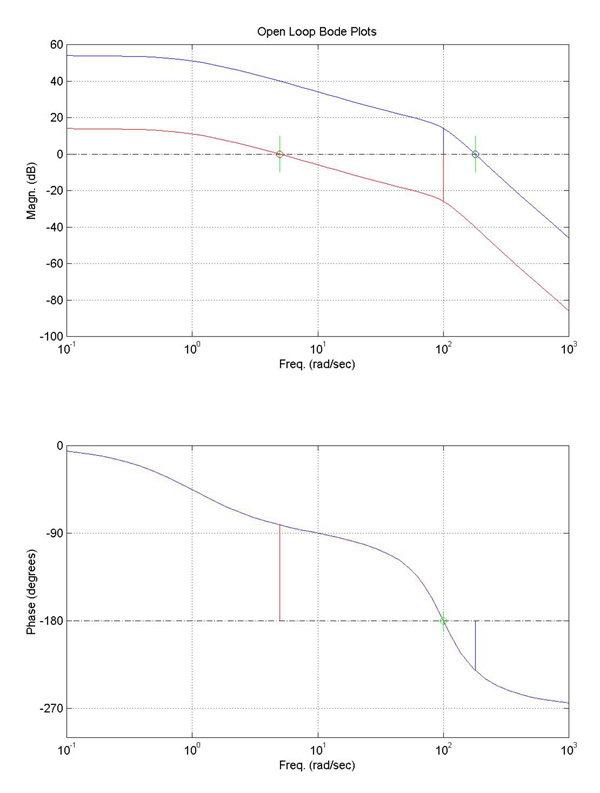Top | Prev | Next

## Stability Criteria - (Gain Margin and Phase Margin)

Think of both of these as safety margins for an open-loop system which you would like to make closed-loop.
• That is, if you are walking next to a cliff, you want a positive space or "margin" of safety between you and a big disaster.
- Hopefully, that intuition may help keep you straight how gain and phase margins are defined -- so that positive margins indicate there is still a safety margin (before instability).
• Conversely, negative margins in an open-loop system indicate instability issues if you try to close this loop!!
 Let's define each, using the figure at right as an aide: GAIN MARGIN - Find the frequency where the PHASE becomes -180 degrees. --- On our picture, this is at 100 (rad/sec) (marked with a green 'o' on the lower plot). - Find the GAIN, G (in dB), at this SAME FREQUENCY (from the upper plot). - Then, we define the GAIN MARGIN as: Gain Margin = 0 - G dB (Note that G is in dB here... But you may want to convert between dB and magnitude as a ratio. To covert magnitude, M, to gain in decibels (dB), G, you use G=20*log10(M). To convert G to M, M=10^(G/20)) Gain Margin = 1/M if you are measuring Magnitude (M) as a ratio (not is dB). PHASE MARGIN - Find the frequency where the GAIN is 0 dB. (This means the output and input amplitudes (magnitudes) are identical at this particular frequency; on the Bode plot, it's where the transfer function crosses 0 dB on the upper [magnitude] plot.) --- For the red Bode plot, this happens at about 5 (rad/sec) [marked with a red 'o' in the upper plot]. --- For the blue Bode plot, the 0 dB crossover occurs at a frequency of around 181 (rad/sec) and is shown with a blue 'o'. - Find the PHASE, P (in degrees), at this SAME FREQUENCY (by now looking at the lower plot). (This particular phase is marked in the lower plot at right for both the red and blue transfer functions with lines of corresponding color...) - Then, we define the PHASE MARGIN as: Phase Margin = +P + 180 degreesNow, to check your understanding, let's solve for the Gain and Phase Margin for both the blue and red transfer functions plotted above. (Note that the BLUE TF was the one shown on the previous page, which we found to be unstable when we 'closed the loop'. The RED TF here is just (1/100) times the BLUE TF.

By choosing a lower gain, we have an open-loop system which will be STABLE when we close the loop.
 - points picked from Bode plots above... Phase Margin =+P + 180 Gain Margin =0 - G For red tf: P = -81.3 degrees (at crossover freq. of 5 (rad/sec)). G = -25.9 dB (at freq of 100 (rad/sec)). +98.7 degrees +25.9 dB For blue tf: P = -231.0 degrees (at crossover freq. of 181 (rad/sec)). G = +14.1 dB (at freq of 100 (rad/sec)). -50.0 degrees -14.1 dB

 gonzo@mit.edu 2.010 Tutorial #3, 29-Sep-00